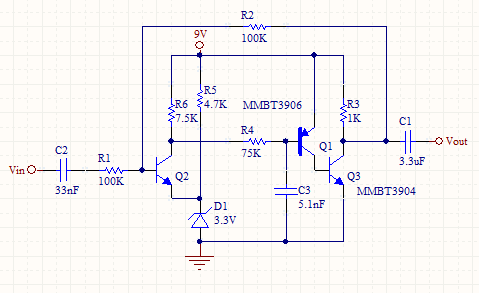# Discrete Transistor AC Op-AMP Circuit

This circuit is an inverting discrete transistor ac op-amp. It doesn't pass DC signals. The gain is set by the feedback resistor R2 and input resistor R1. The transistor amp is a very high gain amplifier. D1 is a bias Diode. So the base of Q2, and collector of Q3 is biased at about 4V. The low pass filter R4 and C3 is a compensation network to stop oscillations. C2 and C1 block DC and pass AC through the circuit.

Since the the circuit has such high gain, the base of Q2 looks like a virtual AC ground, because Vout/infinity= 0V. Thus, any AC current flowing in R1 also flows through R2.

IR2= Vin/R1.

Vout/R2= -IR2.

Thus

Vout/Vin = -R2/R1Transistor AC OP-AMP

Check out our Op-Amp Calculator and Resistor Calculator.

### Equations:

Vout/Vin= -(R2/R1)